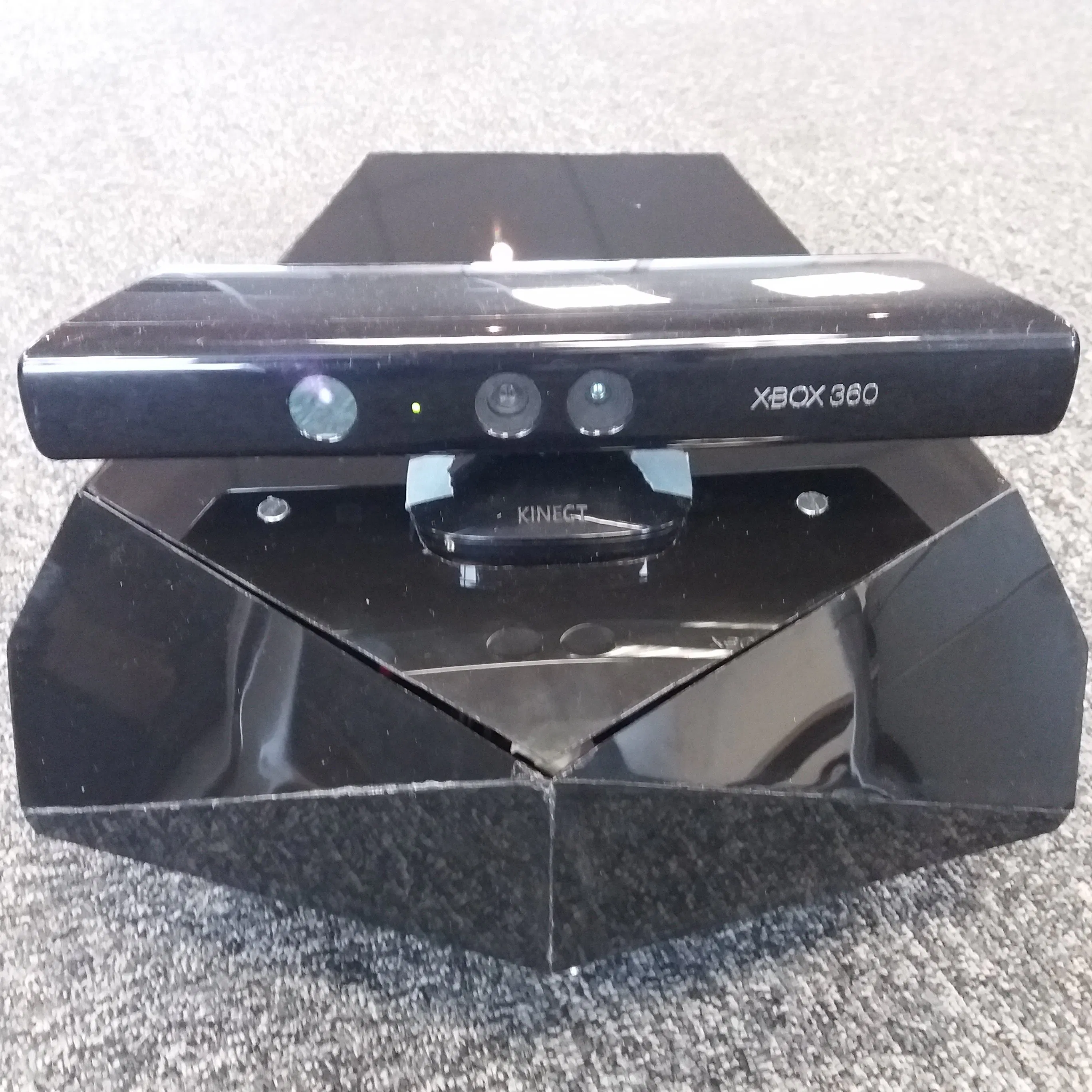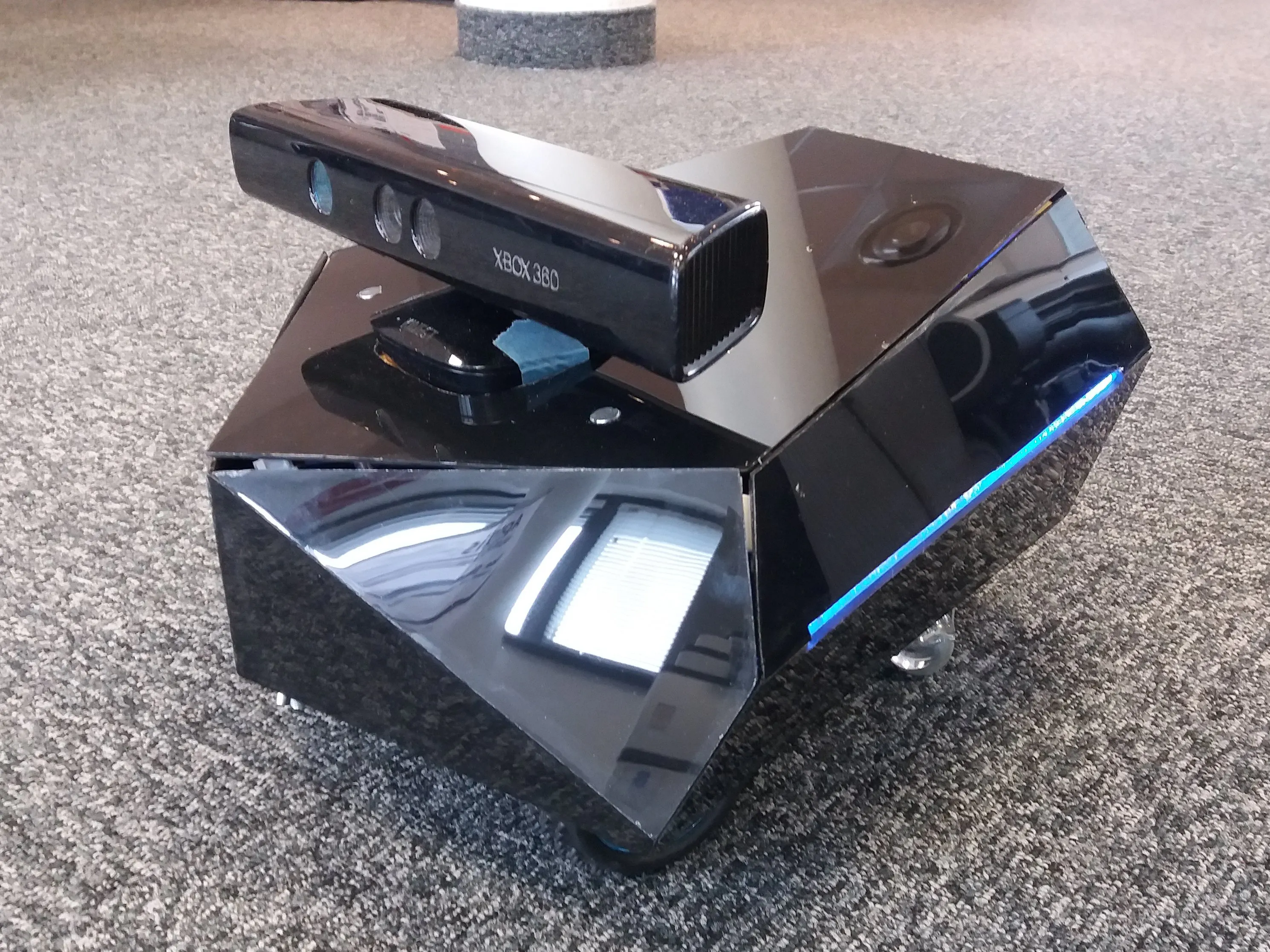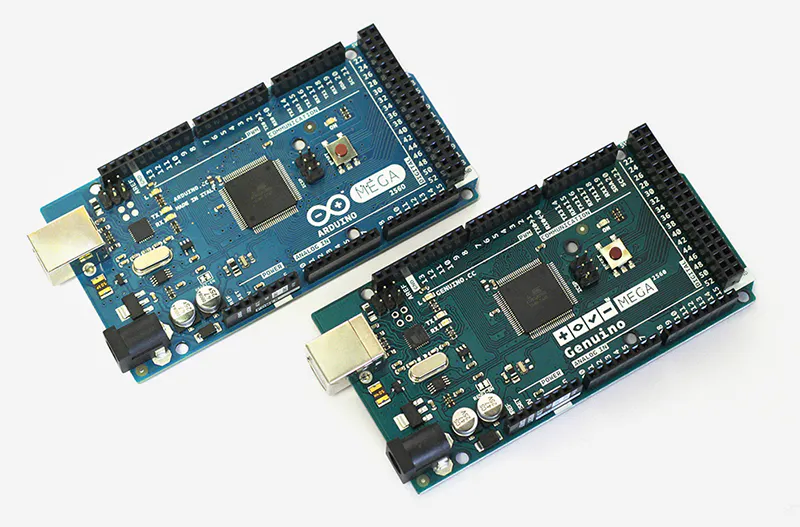# Nox - A House Wandering Robot (ROS)

Nox is a nice (and time-consuming) robot which uses SLAM (ROS) with a Kinect to navigate in its environment.## Things used in this project

### Hardware componentsRaspberry Pi 3 Model B
×1Arduino Mega 2560 & Genuino Mega 2560
×1
 Adafruit Motor/Stepper/Servo Shield for Arduino v2 Kit - v2.3
×1Microsoft Kinect Sensor
×1
 Geared 12V DC Motors with Encoders
×1
 DC-DC Boost Buck Adjustable Step-Up Step-Down Automatic Converter XL6009 Module
×1
 DC-DC Buck Converter 10A
×1

### Software apps and online servicesROS Robot Operating SystemArduino IDE

### Hand tools and fabrication machinesSoldering iron (generic)

## Code

### Arduino motor controller

Arduino
```#include <Adafruit_MotorShield.h>
#include <Wire.h>
#include <PID_v1.h>
#include <ros.h>
#include <std_msgs/String.h>
#include <geometry_msgs/Vector3Stamped.h>
#include <geometry_msgs/Twist.h>
#include <ros/time.h>

//initializing all the variables
#define LOOPTIME                      100     //Looptime in millisecond
const byte noCommLoopMax = 10;                //number of main loops the robot will execute without communication before stopping
unsigned int noCommLoops = 0;                 //main loop without communication counter

char log_msg;
char result;
double speed_cmd_left2 = 0;

const int PIN_ENCOD_A_MOTOR_LEFT = 2;               //A channel for encoder of left motor
const int PIN_ENCOD_B_MOTOR_LEFT = 4;               //B channel for encoder of left motor

const int PIN_ENCOD_A_MOTOR_RIGHT = 3;              //A channel for encoder of right motor
const int PIN_ENCOD_B_MOTOR_RIGHT = 5;              //B channel for encoder of right motor

const int PIN_SIDE_LIGHT_LED = 46;                  //Side light blinking led pin

unsigned long lastMilli = 0;
const double wheelbase = 0.187;               //Wheelbase, in m

double speed_req = 0;                         //Desired linear speed for the robot, in m/s
double angular_speed_req = 0;                 //Desired angular speed for the robot, in rad/s

double speed_req_left = 0;                    //Desired speed for left wheel in m/s
double speed_act_left = 0;                    //Actual speed for left wheel in m/s
double speed_cmd_left = 0;                    //Command speed for left wheel in m/s

double speed_req_right = 0;                   //Desired speed for right wheel in m/s
double speed_act_right = 0;                   //Actual speed for right wheel in m/s
double speed_cmd_right = 0;                   //Command speed for right wheel in m/s

const double max_speed = 0.4;                 //Max speed in m/s

int PWM_leftMotor = 0;                     //PWM command for left motor
int PWM_rightMotor = 0;                    //PWM command for right motor

// PID Parameters
const double PID_left_param[] = { 0, 0, 0.1 }; //Respectively Kp, Ki and Kd for left motor PID
const double PID_right_param[] = { 0, 0, 0.1 }; //Respectively Kp, Ki and Kd for right motor PID

volatile float pos_left = 0;       //Left motor encoder position
volatile float pos_right = 0;      //Right motor encoder position

PID PID_leftMotor(&speed_act_left, &speed_cmd_left, &speed_req_left, PID_left_param, PID_left_param, PID_left_param, DIRECT);          //Setting up the PID for left motor
PID PID_rightMotor(&speed_act_right, &speed_cmd_right, &speed_req_right, PID_right_param, PID_right_param, PID_right_param, DIRECT);   //Setting up the PID for right motor

Adafruit_DCMotor *leftMotor = AFMS.getMotor(1);      //Create left motor object
Adafruit_DCMotor *rightMotor = AFMS.getMotor(2);     //Create right motor object

ros::NodeHandle nh;

//function that will be called when receiving command from host
void handle_cmd (const geometry_msgs::Twist& cmd_vel) {
noCommLoops = 0;                                                  //Reset the counter for number of main loops without communication

speed_req = cmd_vel.linear.x;                                     //Extract the commanded linear speed from the message

angular_speed_req = cmd_vel.angular.z;                            //Extract the commanded angular speed from the message

speed_req_left = speed_req - angular_speed_req*(wheelbase/2);     //Calculate the required speed for the left motor to comply with commanded linear and angular speeds
speed_req_right = speed_req + angular_speed_req*(wheelbase/2);    //Calculate the required speed for the right motor to comply with commanded linear and angular speeds
}

ros::Subscriber<geometry_msgs::Twist> cmd_vel("cmd_vel", handle_cmd);   //create a subscriber to ROS topic for velocity commands (will execute "handle_cmd" function when receiving data)
geometry_msgs::Vector3Stamped speed_msg;                                //create a "speed_msg" ROS message
ros::Publisher speed_pub("speed", &speed_msg);                          //create a publisher to ROS topic "speed" using the "speed_msg" type

const int lightIncNumber = 30;                                                                                                                                       //Number of lightIncrements for side light blinking
int lightInc = 0;                                                                                                                                                    //Init increment for side light blinking
int lightValue [lightIncNumber]= { 10, 40, 80, 160, 255, 255, 255, 255, 255, 255, 255, 255, 255, 255, 255, 255, 255, 255, 160, 80, 40, 10, 0, 0, 0, 0, 0, 0, 0, 0 }; //side light increment values
int lightValueNoComm = { 255, 0, 255, 0, 255, 0, 0, 0, 0, 0, 0, 0, 0, 0, 0, 0, 0, 0, 0, 0, 0, 0, 0, 0, 0 }; //side light increment values
int lightT = 0; //init light period

//__________________________________________________________________________

void setup() {

pinMode(PIN_SIDE_LIGHT_LED, OUTPUT);      //set pin for side light leds as output
analogWrite(PIN_SIDE_LIGHT_LED, 255);     //light up side lights

nh.initNode();                            //init ROS node
nh.getHardware()->setBaud(57600);         //set baud for ROS serial communication
nh.subscribe(cmd_vel);                    //suscribe to ROS topic for velocity commands
nh.advertise(speed_pub);                  //prepare to publish speed in ROS topic

AFMS.begin();

//setting motor speeds to zero
leftMotor->setSpeed(0);
leftMotor->run(BRAKE);
rightMotor->setSpeed(0);
rightMotor->run(BRAKE);

//setting PID parameters
PID_leftMotor.SetSampleTime(95);
PID_rightMotor.SetSampleTime(95);
PID_leftMotor.SetOutputLimits(-max_speed, max_speed);
PID_rightMotor.SetOutputLimits(-max_speed, max_speed);
PID_leftMotor.SetMode(AUTOMATIC);
PID_rightMotor.SetMode(AUTOMATIC);

// Define the rotary encoder for left motor
pinMode(PIN_ENCOD_A_MOTOR_LEFT, INPUT);
pinMode(PIN_ENCOD_B_MOTOR_LEFT, INPUT);
digitalWrite(PIN_ENCOD_A_MOTOR_LEFT, HIGH);                // turn on pullup resistor
digitalWrite(PIN_ENCOD_B_MOTOR_LEFT, HIGH);
attachInterrupt(0, encoderLeftMotor, RISING);

// Define the rotary encoder for right motor
pinMode(PIN_ENCOD_A_MOTOR_RIGHT, INPUT);
pinMode(PIN_ENCOD_B_MOTOR_RIGHT, INPUT);
digitalWrite(PIN_ENCOD_A_MOTOR_RIGHT, HIGH);                // turn on pullup resistor
digitalWrite(PIN_ENCOD_B_MOTOR_RIGHT, HIGH);
attachInterrupt(1, encoderRightMotor, RISING);
}

//_________________________________________________________________________

void loop() {
nh.spinOnce();
if((millis()-lastMilli) >= LOOPTIME)
{                                                                           // enter timed loop
lastMilli = millis();

if (!nh.connected()){
analogWrite(PIN_SIDE_LIGHT_LED, lightValueNoComm[lightInc]);
lightInc=lightInc+1;
if (lightInc >= 25){
lightInc=0;
}
}
else{
analogWrite(PIN_SIDE_LIGHT_LED, lightValue [lightInc]);
lightT = 3000 - ((2625/max_speed)*((abs(speed_req_left)+abs(speed_req_right))/2));
lightInc=lightInc+(30/(lightT/LOOPTIME));
if (lightInc >= lightIncNumber){
lightInc=0;
}
}

if (abs(pos_left) < 5){                                                   //Avoid taking in account small disturbances
speed_act_left = 0;
}
else {
speed_act_left=((pos_left/990)*2*PI)*(1000/LOOPTIME)*radius;           // calculate speed of left wheel
}

if (abs(pos_right) < 5){                                                  //Avoid taking in account small disturbances
speed_act_right = 0;
}
else {
speed_act_right=((pos_right/990)*2*PI)*(1000/LOOPTIME)*radius;          // calculate speed of right wheel
}

pos_left = 0;
pos_right = 0;

speed_cmd_left = constrain(speed_cmd_left, -max_speed, max_speed);
PID_leftMotor.Compute();                                                 // compute PWM value for left motor
PWM_leftMotor = constrain(((speed_req_left+sgn(speed_req_left)*0.0882)/0.00235) + (speed_cmd_left/0.00235), -255, 255); //

if (noCommLoops >= noCommLoopMax) {                   //Stopping if too much time without command
leftMotor->setSpeed(0);
leftMotor->run(BRAKE);
}
else if (speed_req_left == 0){                        //Stopping
leftMotor->setSpeed(0);
leftMotor->run(BRAKE);
}
else if (PWM_leftMotor > 0){                          //Going forward
leftMotor->setSpeed(abs(PWM_leftMotor));
leftMotor->run(BACKWARD);
}
else {                                               //Going backward
leftMotor->setSpeed(abs(PWM_leftMotor));
leftMotor->run(FORWARD);
}

speed_cmd_right = constrain(speed_cmd_right, -max_speed, max_speed);
PID_rightMotor.Compute();                                                 // compute PWM value for right motor
PWM_rightMotor = constrain(((speed_req_right+sgn(speed_req_right)*0.0882)/0.00235) + (speed_cmd_right/0.00235), -255, 255); //

if (noCommLoops >= noCommLoopMax) {                   //Stopping if too much time without command
rightMotor->setSpeed(0);
rightMotor->run(BRAKE);
}
else if (speed_req_right == 0){                       //Stopping
rightMotor->setSpeed(0);
rightMotor->run(BRAKE);
}
else if (PWM_rightMotor > 0){                         //Going forward
rightMotor->setSpeed(abs(PWM_rightMotor));
rightMotor->run(FORWARD);
}
else {                                                //Going backward
rightMotor->setSpeed(abs(PWM_rightMotor));
rightMotor->run(BACKWARD);
}

if((millis()-lastMilli) >= LOOPTIME){         //write an error if execution time of the loop in longer than the specified looptime
Serial.println(" TOO LONG ");
}

noCommLoops++;
if (noCommLoops == 65535){
noCommLoops = noCommLoopMax;
}

publishSpeed(LOOPTIME);   //Publish odometry on ROS topic
}
}

//Publish function for odometry, uses a vector type message to send the data (message type is not meant for that but that's easier than creating a specific message type)
void publishSpeed(double time) {
speed_msg.header.stamp = nh.now();      //timestamp for odometry data
speed_msg.vector.x = speed_act_left;    //left wheel speed (in m/s)
speed_msg.vector.y = speed_act_right;   //right wheel speed (in m/s)
speed_msg.vector.z = time/1000;         //looptime, should be the same as specified in LOOPTIME (in s)
speed_pub.publish(&speed_msg);
nh.spinOnce();
}

//Left motor encoder counter
void encoderLeftMotor() {
else pos_left--;
}

//Right motor encoder counter
void encoderRightMotor() {
else pos_right++;
}

template <typename T> int sgn(T val) {
return (T(0) < val) - (val < T(0));
}
```

## Credits

### RobinB

5 projects • 30 followers
Mechatronic Engineer/Project Manager for AGV (Automated Guided Vehicle). UTBM graduate.